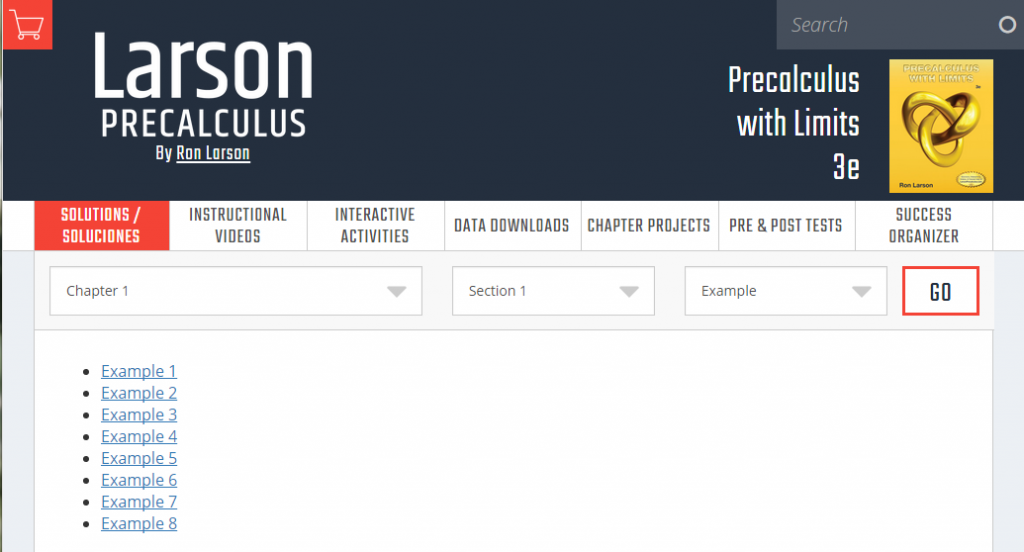# PreCalculus

/PreCalculus
PreCalculus 2019-02-19T15:34:15+00:00

Precalculus delves deeper into the functions and trigonometry you studied in algebra II. Additional topics include matrices and determinants, sequences, series, and probability, analytic geometry, limits and an introduction to calculus.

### September2023

Sun Mon Tue Wed Thu Fri Sat
1
• Polynomial Equations Challenge, Section 2.2 p. 134: 61, 64, 68, 71, 81, 84
2
3
4
5
6
• Section 2.3 p 144 11, 24, 27, 36, , 51, 62, 67, 70
7
• 2.1 - 2.3 Review
8
• Quiz 2.1 - 2.3
9
10
11
• Section 2.4 p. 152 #s 14, 20, 23, 26, 35, 38, 41, 44, 453, 56, 69, 73, 75, 81-84, 95
12
13
• Section 2.5 p. 165 #s 90, 91, 93, 74, 77, 82 no calc
14
15
• Asymptote Review Game
16
17
18
• Section 2.6 p 177: 10-16 even, 26, 29, 33, 60
19
• Review 2.4 - 2.6
20
• Review ws
21
• Chapter 2 Test, Review Rules of Exponents, Radicals p. A23 9-13 odd, 21-29 o, 45-51 o, 69, 71
22
• Understanding Credit Calculations by Hand
23
24
25
26
27
• Section 3.1 Page 208: 13­-16, 19, 21, 22 no calc, 23­-26, 51-­54, 63, 64, 66, 68
28
• 3.2 Two Points & an Asymptote Logs Practice
29
• 3.1 & 3.2 Exponential and Log Practice
30

Use the calendar above to keep track of assignments and use the included links to access on-line material and resources. Subscribe to the calendar using ical

For iPhone:

or Google calendar – Calendar ID:

There are student resources available online at http://larsonprecalculus.com/pcwl3e/. Each example in the text book is worked out via a video. Instructional videos, interactive activities, and data downloads are accessible via this site, as are practice tests and a success organizer for taking notes.Exponential Functions –  Exponential functions are defined and graphed. Examples of how parent and child exponential functions are related are also demonstrated in the video and text.

Logarithmic Functions – This article/video gives the definition of a logarithmic function, shows how to change between logarithmic and exponential forms, describes properties of logs, demonstrates graphing logs, natural logs.

Properties of Logarithms – The video and text describe the change of base formula for logarithms, the product, quotient, and power properties of logarithms, and how to expand and condense logarithms.

Exponential & Logarithmic Equations – Solve logarithmic and exponential equations using change of base, and inverse operations. Video and written examples included.

Ebola 2014 – Use CDC data on the Ebola outbreak and Excel and TI-83+ graphing calculator to create exponential regression curves of best fit.

Exponential and Logarithmic Models – This page contains a video of examples of exponential growth predictions and images of the problems worked out. It also describes various exponential and logarithmic growth models like Gaussian, exponential decay, and logistics growth.

Partial Fractions – The video describes how to write a rational expression as the sum of two or more partial fractions. Five examples are demonstrated including fraction that require linear numerators.

Find the Inverse of a 3×3 Matrix Using a Graphing Calculator – One method of finding the inverse of a 3×3 matrix involves using a graphing calculator.

Linear Regressions on a TI Graphing Calculator – Use a TI graphing calculator to perform a linear regression on data regarding bird wing length and wing beat rate.

Ebola 2014 Revisited – updated data that now points to a logistics curve

Angular to Linear Velocity – watch clips of a bike at night to figure out how to determine the linear speed of the bike